# ISEE Upper Level Quantitative : Data Analysis

## Example Questions

### Example Question #13 : How To Find The Answer From A Table

A fair coin is tossed five times. Which is the greater quantity?

(a) The probability that heads appear four or five times

(b) The probability that heads appear zero or one time

It is impossible to tell from the information given.

(b) is greater.

(a) is greater.

(a) and (b) are equal.

(a) and (b) are equal.

Explanation:

The event in (b) - that heads appear zero or one time - is exactly the same as the event that tails appear four or five times. Since heads and tails come up in each trial with equal probability, the events in (a) and (b) will be equally probable. This makes the quantities equal.

### Example Question #14 : How To Find The Answer From A Table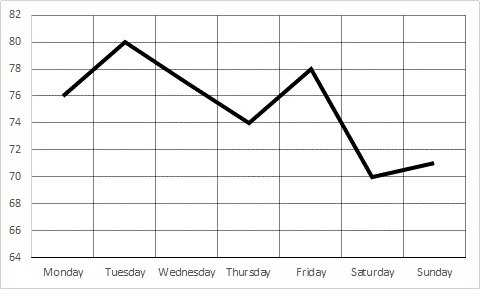Refer to the above graph, which shows the high temperatures in the town of Smithville over seven days.

Which is the greater quantity?

(a) The low temperature on Thursday

(b) The low temperature on Saturday

It is impossible to tell from the information given.

(a) and (b) are equal.

(a) is greater.

(b) is greater.

It is impossible to tell from the information given.

Explanation:

The graph gives absolutely no information about the low temperatures on any of the seven days; the high temperatures have no relevance to the answer in this question.

### Example Question #33 : Data Analysis And Probability

The following is a portion of the menu at Moonbucks Coffee: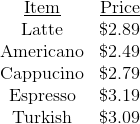Which is the greater quantity?

(a) The price of a latte, a Cafe Americano, an espresso, and a Turkish coffee

(b) The price of a latte, a cappucino, a medium cola, and an espresso

(a) and (b) are equal.

(a) is greater.

It is impossible to tell from the information given.

(b) is greater.

It is impossible to tell from the information given.

Explanation:

The order in choice (b) includes a medium cola, the price of which is not given. It is therefore impossible to tell which order costs more.

### Example Question #15 : How To Find The Answer From A TableRefer to the above graph, which shows the high temperatures in the town of Smithville over seven days.

Which is the greater quantity?

(a) The decrease in the peak temperature between Tuesday and Wednesday

(b) The decrease in the peak temperature between Wednesday and Thursday

(b) is greater.

(a) and (b) are equal.

It is impossible to tell from the information given.

(a) is greater.

(a) and (b) are equal.

Explanation:

Observe the portions of the line graph between Tuesday and Wednesday and between Wednesday and Thursday. The portions have the same slope, so the decreases are the same.

### Example Question #35 : Data Analysis And Probability

Below is a table that gives the population of Washington City for five census years.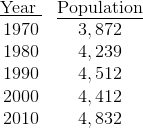Which is the greater quantity?

(a) The population increase in Washington City from 1970 to 1975

(b) The population increase in Washington City from 1975 to 1980

(b) is greater

It is impossible to tell from the information given

(a) and (b) are equal

(a) is greater

It is impossible to tell from the information given

Explanation:

No information is given in the table about the population of Washington City in 1975. Therefore, it cannot be determined with any certainty which population change was the greater.

### Example Question #21 : How To Find The Answer From A Table

Two standard decks of cards are altered as follows: the jacks from Deck 1 are switched with the aces from Deck 2.

Which is the greater quantity?

(a) The probability that a card randomly drawn from Deck 1 is black

(b) The probability that a card randomly drawn from Deck 2 is black

(a) is greater

It is impossible to tell from the information given

(b) is greater

(a) and (b) are equal

(a) and (b) are equal

Explanation:

Two black cards and two red cards from Deck 1 are being switched with two black cards and two red cards from Deck 2, so even after the switch, in each deck, half the cards are black and half are red. Therefore, the probability of drawing a black card from Deck 1 remains, as does that of drawing a black card from Deck 2.

### Example Question #22 : How To Find The Answer From A Table

Two standard decks of cards are altered as follows: two jacks from Deck 1 are switched with two deuces from Deck 2.

Which is the greater quantity?

(a) The probability that a card randomly drawn from Deck 1 is black

(b) The probability that a card randomly drawn from Deck 2 is black

It is impossible to tell from the information given

(a) is greater

(a) and (b) are equal

(b) is greater

It is impossible to tell from the information given

Explanation:

The colors of the cards are not given, so it is now impossible to determine which deck has more black cards than red cards, if either does. For example, if the jacks are black and the deuces are red, Deck 1 now has more red cards and Deck 2 has more black cards; if the reverse holds true of the jacks and the deuces, the reverse holds true of the makeup of the decks.

Therefore, it is unknown whether you are more likely to draw a black card from Deck 1 or Deck 2.

### Example Question #31 : Data Analysis And Probability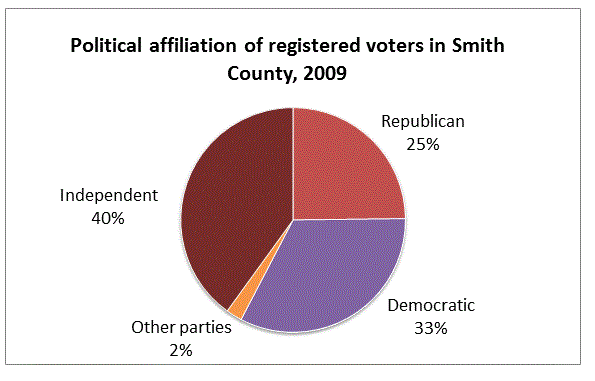Refer to the above diagram. You are given that there are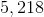registered Democrats in Smith County. Which is the greater quantity?

(a) The number of registered Republicans in Smith County

(b)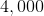(a) is greater.

(b) is greater.

It is impossible to tell from the information given.

(a) and (b) are equal.

(b) is greater.

Explanation:

Letbe the number of Republicans in Smith County. The ratio of Republicans to Democrats is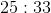; since there areDemocrats, we can set up a proportion as follows: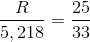Solve for: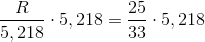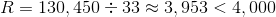There are fewer thanRepublicans in Smith County, so (b) is the greater quantity.

### Example Question #32 : Data Analysis And Probability

Below is a table that gives the population of Washington City for five census years.It is known that 12% of the residents of Washington City were Black in 1970, and that 22% of the residents of Washington City were Black in 2010.

Which is the greater quantity?

(a) The number of residents of Washington City who were not Black in 1970

(b) The number of residents of Washington City who were not Black in 2010

(a) is greater

It cannot be determined from the information given

(a) and (b) are equal

(b) is greater

(b) is greater

Explanation:

(a) 12% of the 3,872 residents of Washington City were Black in 1970. Therefore, 88% of the residents were not Black; this makes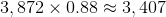residents.

(b) 22% of the 4,832 residents of Washington City were Black in 2010. Therefore, 78% of the residents were not Black; this makes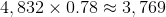residents.

(b) is greater.

### Example Question #31 : Data Analysis

A standard deck of cards is altered by removing two jacks. A card is drawn at random from this altered deck. Which is the greater quantity?

(a) The probability of drawing a red card

(b) The probability of drawing a black card

(a) and (b) are greater

(a) is greater

It is impossible to tell from the information given

(b) is greater